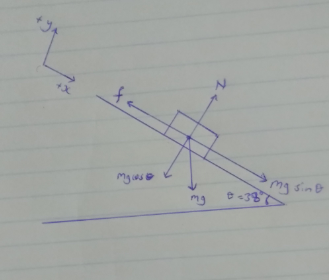# Problem: A 23 kg child goes down a straight slide inclined 38 degrees above horizontal. The child is acted on by his weight, the normal force from the slide, and the kinetic friction. (a) Draw a free body diagram of the child. (b) How large is the normal force of the slide on the child?

###### FREE Expert Solution

(a)88% (118 ratings)###### Problem Details

A 23 kg child goes down a straight slide inclined 38 degrees above horizontal. The child is acted on by his weight, the normal force from the slide, and the kinetic friction.

(a) Draw a free body diagram of the child.

(b) How large is the normal force of the slide on the child?